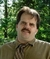## Fill in a varB from code into a cell on Excel spreadsheetTags:
Microsoft Excel
VBA
I have a workbook running a macros for multiple "If Then" statements. One of the IF Then statements in the Macros determines a varB, then that varB is used to calculate another value. How do I then ask the new varB to fill into a blank cell on the Excel spreadsheet?

Thanks. We'll let you know when a new response is added.

It’s been a while but try something like this

```If Range("B65") = "Breast Milk" Then
If Range("C65") = 20 Then varB = 1.41
ElseIf Range("C65") = 22 Then varB = 1.88
ElseIf Range("C65") = 24 Then varB = 2.35
End If
ElseIf Range("B65") = "SSC" Then
varB = 3
ElseIf Range("B65") = "SSC HPF" Then
varB = 3.5
ElseIf Range("B65") = "Alimentum" Then
If Range("C65") = 20 Then varB = 2.7
ElseIf Range("C65") = 22 Then varB = 2.97
ElseIf Range("C65") = 24 Then varB = 3.24
End If
ElseIf Range("B65") = "Neosure" Then
If Range("C65") = 22 Then varB = 2.8
ElseIf Range("C65") = 24 Then varB = 3.05
End If
ElseIf Range("B65") = "Elecare" Then
If Range("C65") = 20 Then varB = 2.06
ElseIf Range("C65") = 22 Then varB = 2.28
ElseIf Range("C65") = 24 Then varB = 2.48
End If
ElseIf Range("B65") = "Similac" Then
If Range("C65") = 20 Then varB = 2.07
ElseIf Range("C65") = 22 Then varB = 2.29
ElseIf Range("C65") = 24 Then varB = 2.49
End If
End If
varB = (varB * Range("C59")) / (Range("B36") * 100)```

## Discuss This Question: 3 Replies

Thanks. We'll let you know when a new response is added.
•Have you written additional macro in this regard? What version that this macro written? Did you got this one from someone?
report
•If possible, post your macro so we can better understand the complete logic you are looking for. Also Let us know what version of Excel you are using.
report
• Thank you! I am new to excel coding and have taught myself through this project I'm working on at work. This is my fist time ever working with macros and I have figured out the coding to this point for the logic that I want to run. I don't know what you mean by "did I get this one from someone?" I had a version of this that I have greatly expanded on to create the worksheet I am developing...
This is the code I'm working with in Excel version 2013:

If Range("B65") = "Breast Milk" Then
If Range("C65") = 20 Then varB = 1.41
If Range("C65") = 22 Then varB = 1.88
If Range("C65") = 24 Then varB = 2.35
ElseIf Range("B65") = "SSC" Then
varB = 3
ElseIf Range("B65") = "SSC HPF" Then
varB = 3.5
ElseIf Range("B65") = "Alimentum" Then
If Range("C65") = 20 Then varB = 2.7
If Range("C65") = 22 Then varB = 2.97
If Range("C65") = 24 Then varB = 3.24
ElseIf Range("B65") = "Neosure" Then
If Range("C65") = 22 Then varB = 2.8
If Range("C65") = 24 Then varB = 3.05
ElseIf Range("B65") = "Elecare" Then
If Range("C65") = 20 Then varB = 2.06
If Range("C65") = 22 Then varB = 2.28
If Range("C65") = 24 Then varB = 2.48
ElseIf Range("B65") = "Similac" Then
If Range("C65") = 20 Then varB = 2.07
If Range("C65") = 22 Then varB = 2.29
If Range("C65") = 24 Then varB = 2.49
End If
varB = (varB * Range("C59")) / (Range("B36") * 100)

I would like the varB that is calculated at the end of this code to fill into a cell, D67 on my worksheet. Is this possible and how? I've played with so many variations to get the code to run and I can't seem to figure it out.

Thank you!
report